# NEET Physics Questions Solved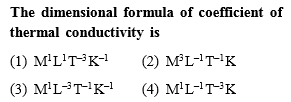(1)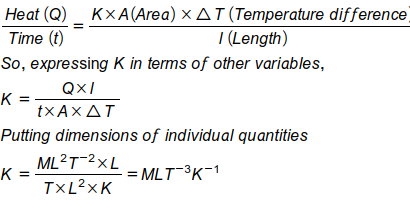Where K is thermal conductivity

Difficulty Level:

• 44%
• 26%
• 24%
• 8%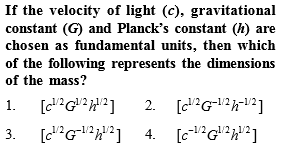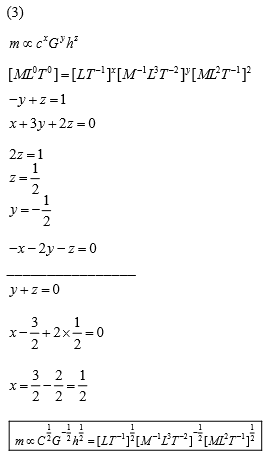Equations are not in right order above. Right order below:

-y + z = 1 ---- (1)

x + 3y  + 2z = 0 ---- (2)

-x -2y -z = 0 ---- (3)

Add (2) and (3) to get say (4)

add (1) and (4) to get z and then get others.

Difficulty Level:

• 22%
• 32%
• 40%
• 9%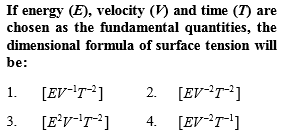Difficulty Level:

• 15%
• 57%
• 19%
• 10%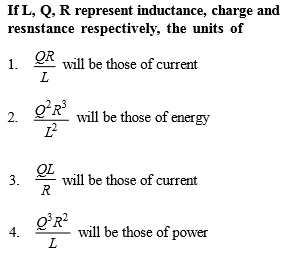[Only for Dropper batch]

Also,

so,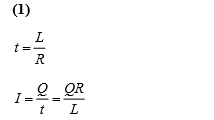Difficulty Level:

• 41%
• 24%
• 27%
• 9%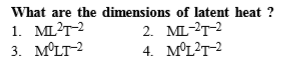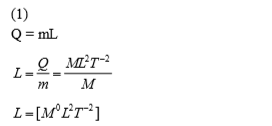Difficulty Level:

• 30%
• 19%
• 19%
• 34%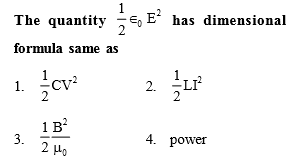[Only for dropper batch]

(3)

Difficulty Level:

• 27%
• 20%
• 38%
• 17%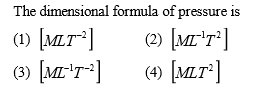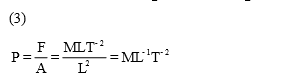Difficulty Level:

• 11%
• 19%
• 67%
• 4%
PMT - 1989

Light year is a unit of

(1) Time

(2) Mass

(3) Distance

(4) Energy

(3) Light year is a distance which light travels in one year.

Difficulty Level:

• 25%
• 6%
• 63%
• 8%

The magnitude of any physical quantity

(1) Depends on the method of measurement

(2) Does not depend on the method of measurement

(3) Is more in SI system than in CGS system

(4) Directly proportional to the fundamental units of mass, length and time

(2) Because magnitude is absolute.

Difficulty Level:

• 27%
• 25%
• 13%
• 36%
PMT - 1990

Which of the following is not equal to watt

(1) Joule/second

(2) Ampere $×$ volt

(3) (Ampere)2 $×$ ohm

(4) Ampere/volt

(4)

Difficulty Level:

• 22%
• 17%
• 30%
• 33%